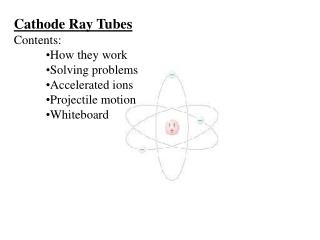DownloadDownload PresentationCathode Ray Tubes Contents: How they work Solving problems Accelerated ions Projectile motion

# Cathode Ray Tubes Contents: How they work Solving problems Accelerated ions Projectile motion

Download Presentation## Cathode Ray Tubes Contents: How they work Solving problems Accelerated ions Projectile motion

- - - - - - - - - - - - - - - - - - - - - - - - - - - E N D - - - - - - - - - - - - - - - - - - - - - - - - - - -
##### Presentation Transcript

1. Cathode Ray Tubes • Contents: • How they work • Solving problems • Accelerated ions • Projectile motion • Whiteboard

2. Cathode Ray Tubes Thermionic emission Accelerated ion (toward + anode) Deflection plates Screen Demo/magnet/VdG TOC

3. Part 1 - acceleration toward the anode: Electrical potential energy =  kinetic energy Ve = 1/2mv2 Example - A CRT uses an accelerating potential of 5200. V. What velocity do the electrons have when they pass through the anode? Ve = 1/2mv2 (5200 V)(1.602x10-19 C) = 1/2(9.11x10-31 kg)v2 v = 4.2765x107 m/s = 4.277x107 m/s (c = 3.00x108 m/s) TOC

4. 20.00 cm 8.00 cm v = 4.2765x107 m/s Part 2 - Steering the electron: What voltage must be applied across the plates above to make the electron emerge from the other end 2.00 cm from the lower plate, assuming it starts parallel to the plates, and 4.00 cm from the lower plate? Which plate would be more positive? Horizontal/vertical>acceleration>force>E Field>Voltage TOC

5. Whiteboards: CRT problems 1 | 2 | 3 | 4 | 5 | 6 TOC

6. 45.0 cm me = 9.11 x 10-31 kg e = 1.602x10-19 C 20.0 cm An electron traveling 114,700 m/s parallel to the plates above, and midway between them is deflected upward by a potential of .0120 V. A. What is the electric field between the plates? E = ΔV/Δx, ΔV = .0120 V, Δx = .200 m E = .0600 V/m W .0600 V/m

7. 45.0 cm me = 9.11 x 10-31 kg e = 1.602x10-19 C 20.0 cm An electron traveling 114,700 m/s parallel to the plates above, and midway between them is deflected upward by a potential of .0120 V. B. What is the electrical force on the electron between the plates? E = F/q, E = .0600 V/m, q = -1.602x10-19 C F = 9.6120x10-21 N = 9.61x10-21 N W 9.61x10-21 N

8. 45.0 cm me = 9.11 x 10-31 kg e = 1.602x10-19 C 20.0 cm An electron traveling 114,700 m/s parallel to the plates above, and midway between them is deflected upward by a potential of .0120 V. C. What is the upward acceleration of the electron between the plates? F = ma, F = 9.6120x10-21 N, m = 9.11x10-31 kg a = 1.0551x1010 m/s/s = 1.06x1010 m/s/s (You can neglect gravity) W 1.06x1010 m/s/s

9. 45.0 cm me = 9.11 x 10-31 kg e = 1.602x10-19 C 20.0 cm An electron traveling 114,700 m/s parallel to the plates above, and midway between them is deflected upward by a potential of .0120 V. D. For what time is the electron between the plates? V = s/t, V = 114,700, s = .45 m t = 3.9233x10-6 s = 3.92x10-6 s W 3.92x10-6 s

10. 45.0 cm me = 9.11 x 10-31 kg e = 1.602x10-19 C 20.0 cm An electron traveling 114,700 m/s parallel to the plates above, and midway between them is deflected upward by a potential of .0120 V. E. What is the vertical displacement of the electron while it passes between the plates? s = ut + 1/2at2, u = 0, t = 3.9233x10-6 s, a = 1.0551x1010 m/s/s s = .0812 m = 8.12 cm W 8.12 cm

11. 45.0 cm me = 9.11 x 10-31 kg e = 1.602x10-19 C 20.0 cm An electron traveling 114,700 m/s parallel to the plates above, and midway between them is deflected upward by a potential of .0120 V. F. Through what potential was the electron accelerated to reach a velocity of 114,700 m/s from rest? Ve = 1/2mv2, q = 1.602x10-19 C, v = 114,700, m = 9.11x10-31 kg V = .0374 V W .0374 V

12. 17.5 cm me = 9.11 x 10-31 kg e = 1.602x10-19 C 10.2 cm • There is an electric field between these plates of 9420 V/m that makes the electrons that enter midway, nearly strike the bottom plate before they emerge from the plates. • What is the voltage across these plates? • What is the force on the electrons between the plates? • What is the downward acceleration of the electrons? • What time is the electron between the plates • What is the horizontal velocity of the electrons? • What voltage accelerated them to this speed before they got here? E = V/d, E = F/q, F = ma, s = ut + 1/2at2, v = s/t, Ve = 1/2mv2, q = 1.602x10-19 C, m = 9.11x10-31 kg W 960.84 V 1.50908E-15N 1.65651E+15m/s/s 7.84698E-09s 22301572.25m/s 1414.15V

13. 5.00 cm x Screen is 8.75 cm from plates 4.00 cm • The electron enters the region between the deflecting plates with a horizontal velocity of 1,450,000 m/s. There is a voltage of 5.66 V across the plates, with the upper plate being positive. Where will the electron hit the screen 8.75 cm from the end of the deflecting plates? Assume that the electric field between the plates is uniform, and that you can neglect gravity. • Hint - find the exit position, and velocity in x and y components. • You will suvat twice E = V/d, E = F/q, F = ma, s = ut + 1/2at2, v = s/t, q = 1.602x10-19 C, m = 9.11x10-31 kg 2.48829E+13m/s/s 3.44828E-08s(twixt plates) 0.014793624m (leaving plates) 858030.2055m/s(up) 6.03448E-08s (to screen) 0.051777685m (twixt plates and screen) 0.066571309 m total W